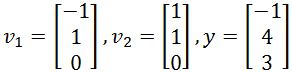# Orthogonal projections

### Orthogonal projections

#### Lessons

The Orthogonal Decomposition Theorem
Let $S$ be a subspace in $\Bbb{R}^n$. Then each vector $y$ in $\Bbb{R}^n$ can be written as:

$y=\hat{y}+z$

where $\hat{y}$ is in $S$ and $z$ is in $S^{\perp}$. Note that $\hat{y}$ is the orthogonal projection of $y$ onto $S$

If {$v_1,\cdots ,v_p$} is an orthogonal basis of $S$, then

proj$_sy=\hat{y}=\frac{y \cdot v_1}{v_1 \cdot v_1}v_1 + \frac{y \cdot v_2}{v_2 \cdot v_2}v_2 + \cdots + \frac{y \cdot v_p}{v_p \cdot v_p}v_p$

However if {$v_1,\cdots ,v_p$} is an orthonormal basis of $S$, then

proj$_sy=\hat{y}=(y \cdot v_1)v_1+(y \cdot v_2)v_2 + \cdots + (y \cdot v_p)v_p$

Property of Orthogonal Projection
If {$v_1,\cdots ,v_p$} is an orthogonal basis for $S$ and if $y$ happens to be in $S$, then
proj$_sy=y$

In other words, if y is in $S=$Span{$v_1,\cdots ,v_p$}, then proj$_S y=y$.

The Best Approximation Theorem
Let $S$ be a subspace of $\Bbb{R}^n$. Also, let $y$ be a vector in $\Bbb{R}^n$, and $\hat{y}$ be the orthogonal projection of $y$ onto $S$. Then $y$ is the closest point in $S$, because

$\lVert y- \hat{y} \rVert$ < $\lVert y-u \rVert$

where $u$ are all vectors in $S$ that are distinct from $\hat{y}$.
• Introduction
Orthogonal Projections Overview:
a)
The Orthogonal Decomposition Theorem
• Make $y$ as the sum of two vectors $\hat{y}$ and $z$
• Orthogonal basis → $\hat{y}= \frac{y \cdot v_1}{v_1 \cdot v_1}v_1 + \cdots + \frac{y \cdot v_p}{v_p \cdot v_p}v_p$
• Orthonormal basis → $\hat{y}=(y\cdot v_1)v_1+\cdots +(y\cdots v_p)v_p$
$z=y - \hat{y}$

b)
Property of Orthogonal Projections
• proj$_s y=y$
• Only works if $y$ is in $S$

c)
The Best Approximation Theorem
• What is the point closest to $y$ in $S$? $\hat{y}$!
• Reason why: $\lVert y - \hat{y} \rVert$ < $\lVert y-u \rVert$
• The Distance between the $y$ and $\hat{y}$

• 1.
The Orthogonal Decomposition Theorem
Assume that {$v_1,v_2,v_3$} is an orthogonal basis for $\Bbb{R}^n$. Write $y$ as the sum of two vectors, one in Span{$v_1$}, and one in Span{$v_2,v_3$}. You are given that: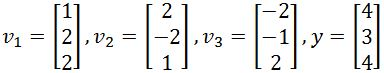• 2.
Verify that {$v_1,v_2$} is an orthonormal set, and then find the orthogonal projection of $y$ onto Span{$v_1,v_2$}.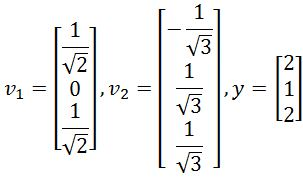• 3.
Best Approximation
Find the best approximation of $y$ by vectors of the form $c_1 v_1+c_2 v_2$, where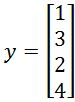, and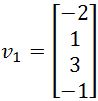,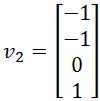.

• 4.
Finding the Closest Point and Distance
Find the closest point to $y$ in the subspace $S$ spanned by $v_1$ and $v_2$.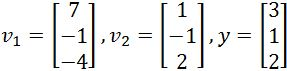• 5.
Find the closest distance from $y$ to $S=$Span{$v_1,v_2$} if